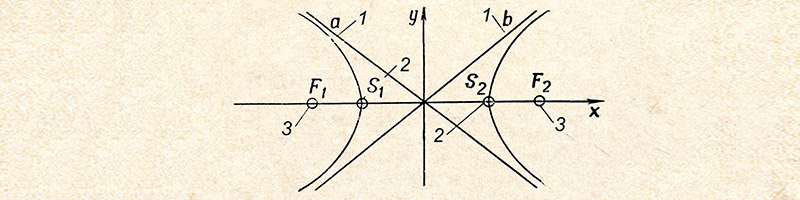# Algebra 2

Often the curriculum in Algebra 2 includes quadratic equations. One of the surprising ways quadratic equations appear in real life is in the design of rollercoasters. Many of the curves and swoops actually reveal parabolas that can be mathematically defined by quadratic equations. If you need to understand the material that you are being taught and it’s practical real life applications, I can help.

#### General Overview of Algebra 2 Content

(Content varies across curricula.)

• Expressions, Equations & Inequalities
• Functions & Graphs: Piecewise, Absolute Value, Correlation & Best-Fitting Lines
• Linear Systems: Solving Graphically & Algebraically with Three Variables, Linear Programming

• Matrices: All Operations, Determinant & Cramer’s Rule, Identity & Inverse Matrices